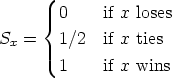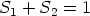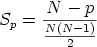## Thursday, June 12, 2008

### Generalizing the ELO Rating System for Multiple Players

The original ELO rating system was designed for two player games. I've had a need to generalize the ELO rating system for games where there are more than two players. I've come with with some modifications to the system to allow for multiple players.

The ELO system assigns a pool of players various ranks based on their abilities. These ranks can be used to estimate the likelihood for a player to win a given game.

For a given game, each player is assigned an estimated score which is roughly equivalent to the chance that the player has of winning the game. After the game, each player's actual score is compared to his estimated score and his rating will be updated.

There are a series of math equations that govern the system.

Let's start with a two player game, and then generalize from there.

For two players with rankings R1 and R2, the estimated scores E1 and E2 are computed:Note that:Once the game is finished, a scoring function determines each player's actual score Sx. A common scoring function for games such as chess where a player x may win, lose, or tie against another opponent is:Note that:Player x's new rating Rx is then calculated:Now we would like to generalize this system for N number of players with ratings R1, R2, ..., RN-1, RN.

We can make the observation that a game with greater than two players is nearly approximated by a set of games where every player is participating in a two-player game with every other opponent.

The number of games this represents is expressed by:The estimated score for a player x Ex can then be computed:Note that:Now we would like a scoring function for the result of a game with more than two players. In a game where each player is assigned a place p (e.g. first, second, third) we might choose the following function:Note that:Finally, every player's actual score is compared to his estimated score and the ratings updated using equation described earlier.

There are two terms D and K that are in the equations above but they have not been explained yet.

The D term is a number that roughly represents the weight given to a player's rating when determining their estimated score. The superiority of a player over another represented by his superior rating will mean less for higher values for D. That is to say that for higher values of D, the fact that one player has a much higher rating an another is less significant in estimating the outcome of a game. In games where luck more influences the outcome of a game than does skill, a higher value of K is more appropriate.

The K term is a factor that scales the magnitude of the change to a player's rating after a given game. A player's rating after a game will change more for higher values of K. Generally, the value of K should be higher when a player's rating is less certain. This may be because the player has not participated in many games. As players play more games and their rating becomes more representative of their skill level, the value of K should be reduced.Anonymous said...

Any improvements on this?